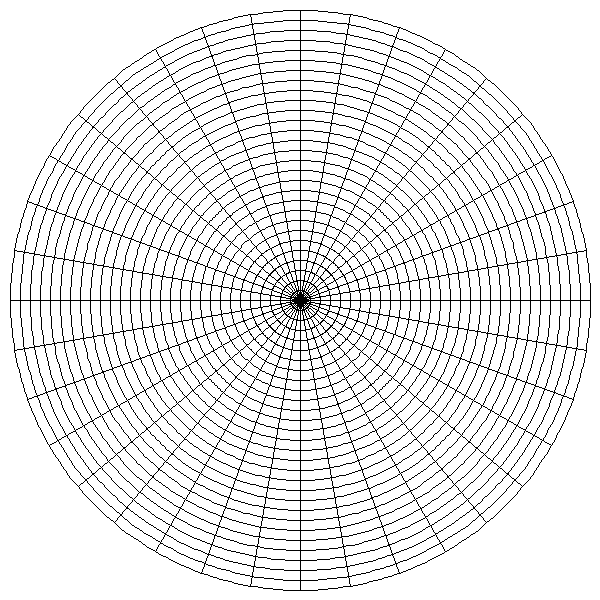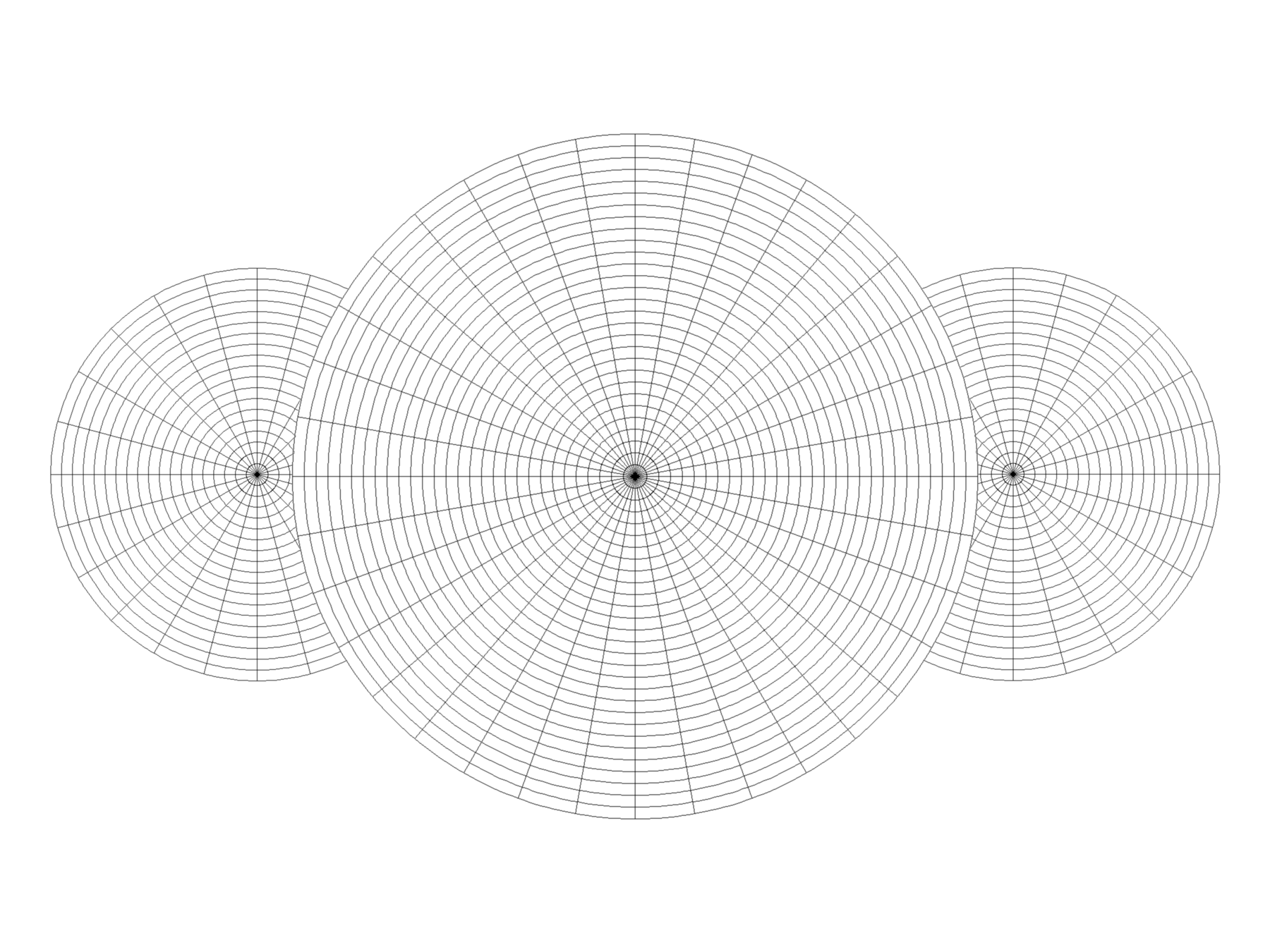# Circular Grids With Python and Pillow

I want a circular grid for drawing. Let's make one with Python!

Post added by

900 words (about 5 minutes to read)

Published

A while back, I wrote about drawing grids with Python and Pillow. I no longer use that code so much, since Procreate now includes square grids in its drawing aid tools.

One idea sitting in my Taskwarrior queue for a full year now would still be useful, though. A circle template could help me break out of the square grid with my Celtic and Tangle drawings.

I already create circular drawings using symmetry tools in my drawing apps. Those are doodles, though: unplanned and improvised. I sketch and see what the automated symmetry produces from my linework. Circle templates simplify planning a complex image which I then produce, probably without using symmetry tools.

So, let’s write a little code!

I’ll keep using Python, since that worked for me last time. Lately I have been using the Anaconda Distribution for my Python programming needs. It includes a number of Python packages, including Pillow!

My template includes three characteristics:

• an origin in the center of my square image
• some concentric circles increasing in radius by a fixed amount
• some line segments slicing the image from the origin point to the outermost circle

## Write some code

I will save myself effort by grabbing some of the work used for drawing grids and putting into a new class.

``````"""
Utility script to draw concentric circle templates for drawing
"""

import argparse

from PIL import Image, ImageDraw

DEFAULT_SIZE = 600
DEFAULT_CIRCLES = 10
DEFAULT_SLICES = 12

class CircleTemplate:
"""
Draws a circle template
"""
def __init__(self, size, circle_count, slice_count):
self.size = size
self.circle_count = circle_count
self.slice_count = slice_count
self.image = Image.new(mode='L', size=(size, size), color=255)

def save(self):
"""Write my circle template image to file"""
filename = "circle-{}-{}-{}.png".format(self.size, self.circle_count, self.slice_count)
print("Saving {}".format(filename))
self.image.save(filename)

def show(self):
"""Display my circle template image on screen"""
self.image.show()

def main():
"""Create a circle template from command line options"""
# Get details from command line or use defaults
parser = argparse.ArgumentParser()
parser.add_argument("--size", help="length of image side in pixels",
type=int, default=DEFAULT_SIZE)
parser.add_argument("--circles", help="number of circles",
type=int, default=DEFAULT_CIRCLES)
parser.add_argument("--slices", help="number of slices",
type=int, default=DEFAULT_SLICES)
args = parser.parse_args()
size = args.size
circle_count = args.circles
slice_count = args.slices
circle_template = CircleTemplate(size, circle_count, slice_count)
circle_template.show()

if __name__ == '__main__':
main()
``````

My `CircleTemplate` class knows how to construct, save, and show a blank image. argparse processes the command line arguments for image size, number of circles, and number of slices. I added defaults so I don’t have to type in a value every time I tested the script for this post.

I can build on this framework. Time to fill in the blanks.

## Draw some circles

``````from PIL import Image, ImageDraw

class CircleTemplate:
"""
Draws a circle template
"""
def __init__(self, size, circle_count, slice_count):
# ...
self.midpoint = int(size / 2)
self._draw()

# ...

def _draw(self):
"""Create circles and slices in-memory"""
draw = ImageDraw.Draw(self.image)
largest_circle = self._draw_circles(draw)
self._draw_slices(draw, largest_circle)
del draw

def _draw_circles(self, draw):
if self.circle_count <= 0:
return 0

radius_step = int(self.midpoint / self.circle_count)

for radius in range(0, self.midpoint, radius_step):
bounding_box = [
(self.midpoint - radius, self.midpoint - radius),
(self.midpoint + radius, self.midpoint + radius)]
draw.arc(bounding_box, 0, 360)
``````

I need to figure out my origin, the center for my circles and slices. Since the image is a square, it will be the same along both the X and Y axes. This means I only need to calculate a single midpoint.

Each time we move on to a new radius, ImageDraw.arc creates a circle by drawing a 360 degree arc within `bounding_box`, a square that extends `radius` pixels from a midpoint along the `x` and `y` axes.Concentric circles

## Add some pie slices

Right. I could do some moderately clever math to calculate angles and draw lines from the midpoint, or I could use the existing ImageDraw.pieslice method to accomplish pretty much the same thing. If you read the section title, you can probably guess what I chose.

``````class CircleTemplate:
# ...
def _draw(self):
"""Create circles and slices in-memory"""
draw = ImageDraw.Draw(self.image)
largest_circle = self._draw_circles(draw)
self._draw_slices(draw, largest_circle)
del draw

def _draw_circles(self, draw):
if self.circle_count <= 0:
return 0

radius_step = int(self.midpoint / self.circle_count)
# To remember the largest circle we drew.
last_radius = 0

for radius in range(0, self.midpoint, radius_step):
bounding_box = [
(self.midpoint - radius, self.midpoint - radius),
(self.midpoint + radius, self.midpoint + radius)]
draw.arc(bounding_box, 0, 360)
last_radius = radius

return last_radius

def _draw_slices(self, draw, radius):
if self.slice_count <= 0:
return

pie_box = [
(self.midpoint - radius, self.midpoint - radius),
(self.midpoint + radius, self.midpoint + radius)]
angle = 360 / self.slice_count
start_angle = 0

for pieslice in range(1, self.slice_count):
end_angle = angle * pieslice
draw.pieslice(pie_box, start_angle, end_angle)
``````

I’m dividing the 360 degrees of a circle into slice_count pieces. `ImageDraw.pieslice` draws a tidy wedge at the angles we give it fitting the bounding box defined by my largest circle.

How does that look?Concentric circles divided by pie slices

It looks pretty cool.

I need more circles and slices for the drawings I’m thinking of, though. Many more.

``````\$ python3 circle_template.py --circles=30 --slices=36
Saving circle-600-30-36.png
``````

Yes, that’s more like it.Generated circle template

This is all I need for a drawing template. Using transformation tools and the right blending modes, I can manuever and manipulate my grid however I need for a drawing template!template prepared for drawing

I’ll stop here so I can get to my drawing.